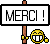[Livre] The Moment-SOS Hierarchy - Lectures in Probability, Statistics, Computational Geometry, Control and Nonlinear PDEs
The Moment-SOS Hierarchy
Lectures in Probability, Statistics, Computational Geometry, Control and Nonlinear PDEs

The moment-SOS hierarchy is a powerful methodology that is used to solve the Generalized Moment Problem (GMP) where the list of applications in various areas of Science and Engineering is almost endless. Initially designed for solving polynomial optimization problems (the simplest example of the GMP), it applies to solving any instance of the GMP whose description only involves semi-algebraic functions and sets. It consists of solving a sequence (a hierarchy) of convex relaxations of the initial problem, and each convex relaxation is a semidefinite program whose size increases in the hierarchy.

The goal of this book is to describe in a unified and detailed manner how this methodology applies to solving various problems in different areas ranging from Optimization, Probability, Statistics, Signal Processing, Computational Geometry, Control, Optimal Control and Analysis of a certain class of nonlinear PDEs. For each application, this unconventional methodology differs from traditional approaches and provides an unusual viewpoint. Each chapter is devoted to a particular application, where the methodology is thoroughly described and illustrated on some appropriate examples.

The exposition is kept at an appropriate level of detail to aid the different levels of readers not necessarily familiar with these tools, to better know and understand this methodology.

[Lire la suite]